SLVSCE1C August   2014  – November 2015

PRODUCTION DATA.

1. Features
2. Applications
3. Description
4. Application Schematic
5. Revision History
6. Pin Configuration and Functions
7. Specifications
8. Parametric Measurement Information
9. Detailed Description
1. 9.1 Overview
2. 9.2 Functional Block Diagram
3. 9.3 Feature Description
4. 9.4 Device Functional Modes
10. 10Applications and Implementation
1. 10.1 Application Information
2. 10.2 Typical Application
1. 10.2.1 Precision Current Limiting and Protection for White Goods
3. 10.3 System Examples
11. 11Power Supply Recommendations
12. 12Layout
13. 13Device and Documentation Support
14. 14Mechanical, Packaging, and Orderable Information

• D|8

## 10 Applications and Implementation

NOTE

Information in the following applications sections is not part of the TI component specification, and TI does not warrant its accuracy or completeness. TI’s customers are responsible for determining suitability of components for their purposes. Customers should validate and test their design implementation to confirm system functionality.

### 10.1 Application Information

The TPS25921x is a smart eFuse. It is typically used for Hot-Swap and Power rail protection applications. It operates from 4.5 V to 18 V with programmable current limit, overvoltage and undervoltage protection. The device aids in controlling the in-rush current and provides precise current limiting during overload conditions for systems such as White Goods, Set-Top-Box, DTVs, Gaming Consoles, SSDs/HDDs and Smart Meters. The device also provides robust protection for multiple faults on the sub-system rail.

Alternatively, the WEBENCH® software may be used to generate a complete design. The WEBENCH® software uses an iterative design procedure and accesses a comprehensive database of components when generating a design. Additionally, a spreadsheet design tool TPS25921 Design Calculator is available on web folder.

### 10.2 Typical Application

#### 10.2.1 Precision Current Limiting and Protection for White Goods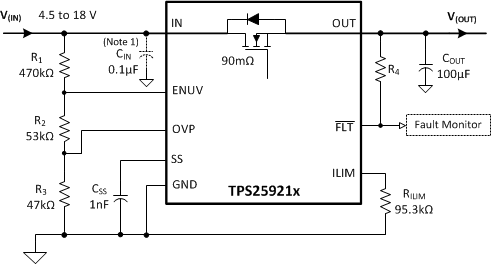1. CIN: Optional and only for noise suppression.
Figure 30. Typical Application Schematics: eFuse for White Goods

#### 10.2.1.1 Design Requirements

For this design example, use the parameters shown in Table 2.

### Table 2. Design Parameters

DESIGN PARAMETER EXAMPLE VALUE
Input voltage range, V(IN) 12 V
Undervoltage lockout set point, V(UV) 8 V
Overvoltage protection set point , V(OV) 17 V
Load at Start-Up , RL(SU) 24 Ω
Current limit, ILIMIT 1 A
Load capacitance , C(OUT) 100 µF
Maximum ambient temperatures , TA 85°C

#### 10.2.1.2 Detailed Design Procedure

The following design procedure can be used to select component values for the TPS25921A and TPS25921L.

#### 10.2.1.2.1 Step by Step Design Procedure

To begin the design process a few parameters must be decided upon. The designer needs to know the following:

• Normal input operation voltage
• Maximum output capacitance
• Maximum current Limit
• Maximum ambient temperature of operation

This design procedure below seeks to control the junction temperature of device under both static and transient conditions by proper selection of output ramp-up time and associated support components. The designer can adjust this procedure to fit the application and design criteria.

#### 10.2.1.2.2 Programming the Current-Limit Threshold: R(ILIM) Selection

The R(ILIM) resistor at the ILIM pin sets the over load current limit, this can be set using Equation 5.

Equation 8.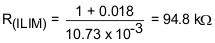Choose closest standard value: 95.3 kΩ, 1% standard value resistor.

#### 10.2.1.2.3 Undervoltage Lockout and Overvoltage Set Point

The undervoltage lockout (UVLO) and overvoltage trip point are adjusted using the external voltage divider network of R1, R2 and R3 as connected between IN, ENUV, OVP and GND pins of the device. The values required for setting the undervoltage and overvoltage are calculated solving Equation 9 and Equation 10.

Equation 9.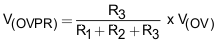Equation 10.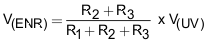For minimizing the input current drawn from the power supply \{I(R123) = V(IN)/(R1 + R2 + R3)\}, it is recommended to use higher values of resistance for R1, R2 and R3.

However, leakage currents due to external active components connected to the resistor string can add error to these calculations. So, the resistor string current, I(R123) must be chosen to be 20x greater than the leakage current expected.

From the device electrical specifications, V(OVPR) = 1.40 V and V(ENR) = 1.40 V. For design requirements, V(OV) is 17 V and V(UV) is 8 V. To solve the equation, first choose the value of R3 = 47 kΩ and use Equation 9 to solve for (R1 + R2) = 523.71 kΩ. Use Equation 10 and value of (R1 + R2) to solve for R2 = 52.88 kΩ and finally R1= 470.83 kΩ.

Using the closest standard 1% resistor values gives R1 = 470 kΩ, R2 = 53 kΩ, and R3 = 47 kΩ.

The power failure threshold is detected on the falling edge of supply. This threshold voltage is 4% lower than the rising threshold, V(UV). This is calculated using Equation 11.

Equation 11. V(PFAIL) = 0.96 x V(UV)

Power fail threshold set is : 7.68 V

#### 10.2.1.2.4 Setting Output Voltage Ramp time (tSS)

For a successful design, the junction temperature of device should be kept below the absolute-maximum rating during both dynamic (start-up) and steady state conditions. Dynamic power stresses often are an order of magnitude greater than the static stresses, so it is important to determine the right start-up time and in-rush current limit required with system capacitance to avoid thermal shutdown during start-up with and without load.

The ramp-up capacitor C(SS) needed is calculated considering the two possible cases:

#### 10.2.1.2.4.1 Case1: Start-up Without Load: Only Output Capacitance C(OUT) Draws Current During Start-up

During start-up, as the output capacitor charges, the voltage difference across the internal FET decreases, and the power dissipated decreases as well. Typical ramp-up of output voltage V(OUT) with inrush current limit of 0.5A and power dissipated in the device during start-up is shown in Figure 31. The average power dissipated in the device during start-up is equal to area of triangular plot (red curve in Figure 32) averaged over tSS.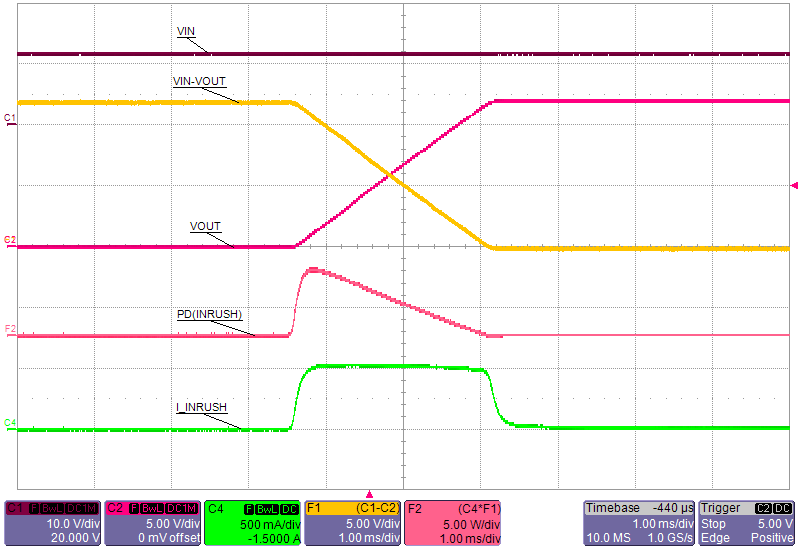V(IN) = 12 V C(SS) = 1 nF C(OUT)=100 µF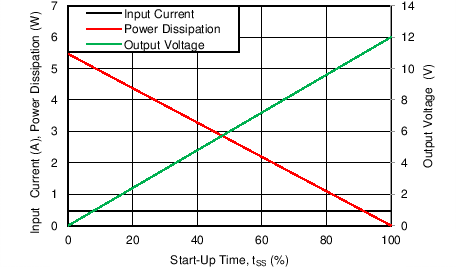V(IN) = 12 V C(SS) = 1 nF C(OUT)=100 µF
Figure 32. PD(INRUSH) Due to Inrush Current

For TPS25921 device, the inrush current is determined as,

Equation 12.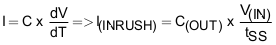Power dissipation during start-up is:

Equation 13.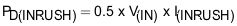Equation 13 assumes that load does not draw any current until the output voltage has reached its final value.

#### 10.2.1.2.4.2 Case 2: Start-up With Load: Output Capacitance C(OUT) and Load Draws Current During Start-up

When load draws current during the turn-on sequence, there will be additional power dissipated. Considering a resistive load RL(SU) during start-up, load current ramps up proportionally with increase in output voltage during tSS time. Typical ramp-up of output voltage, load current and power dissipation in the device is shown in Figure 33 and power dissipation with respect to time is plotted in Figure 34. The additional power dissipation during start-up phase is calculated as follows.

Equation 14.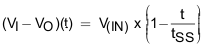Equation 15.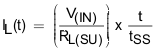Where RL(SU) is the load resistance present during start-up. Average energy loss in the internal FET during charging time due to resistive load is given by:

Equation 16.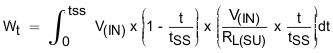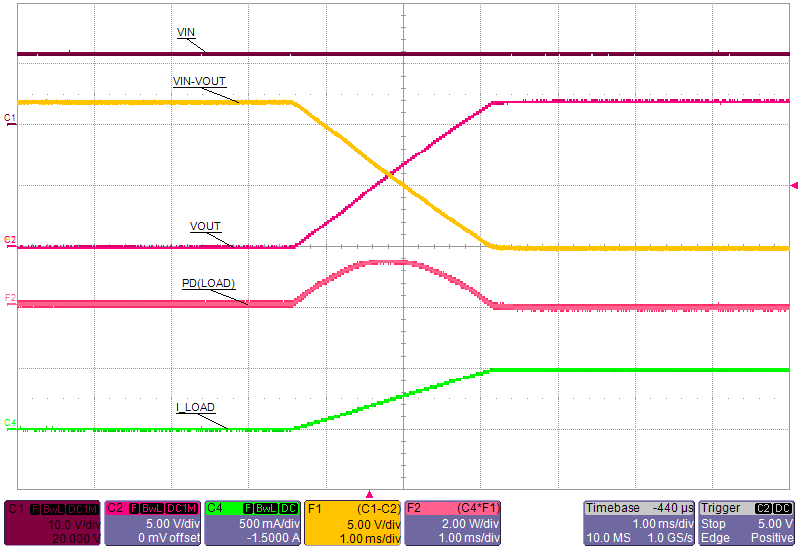V(IN) = 12 V C(SS) = 1 nF , C(OUT)=100 µF RL(SU) = 24 Ω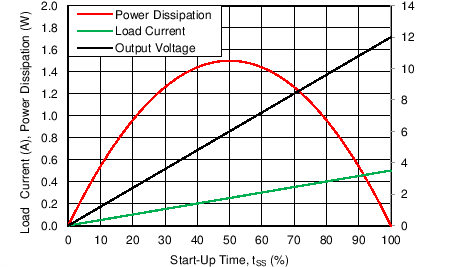V(IN) = 12 V C(SS) = 1 nF RL(SU) = 24 Ω

On solving Equation 16 the average power loss in the internal FET due to load is:

Equation 17.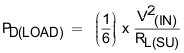Total power dissipated in the device during startup is:

Equation 18.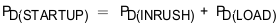Total current during startup is given by:

Equation 19.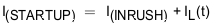If I(STARTUP) > ILIMIT, the device limits the current to ILIMIT and the current limited charging time is determined by:

Equation 20.The power dissipation, with and without load, for selected start-up time should not exceed the shutdown limits as shown in Figure 35.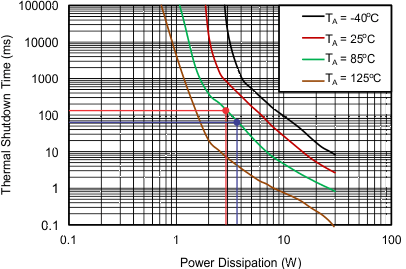Figure 35. Thermal Shutdown Limit Plot

For the design example under discussion,

Select ramp-up capacitor C(SS) = 1nF, using Equation 2.

Equation 21.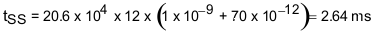The inrush current drawn by the load capacitance (C(OUT)) during ramp-up using Equation 3.

Equation 22.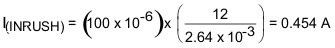The inrush Power dissipation is calculated, using Equation 13.

Equation 23.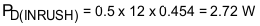For 2.72 W of power loss, the thermal shut down time of the device should not be less than the ramp-up time tSS to avoid the false trip at maximum operating temperature. From thermal shutdown limit graph Figure 35 at
TA = 85°C, for 2.72 W of power the shutdown time is ~170 ms. So it is safe to use 2.64 ms as start-up time without any load on output.

Considering the start-up with load 24 Ω, the additional power dissipation, when load is present during start up is calculated, using Equation 17.

Equation 24.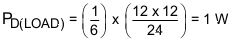The total device power dissipation during start up is:

Equation 25.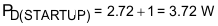From thermal shutdown limit graph at TA = 85°C, the thermal shutdown time for 3.72 W is close to 60 ms. It is safe to have 30% margin to allow for variation of system parameters such as load, component tolerance, and input voltage. So it is well within acceptable limits to use the 1 nF capacitor with start-up load of 24 Ω.

If there is a need to decrease the power loss during start-up, it can be done with increase of C(SS) capacitor.

To illustrate, choose C(SS) = 4.7 nF as an option and recalculate:

Equation 26.Equation 27.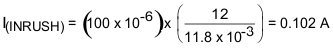Equation 28.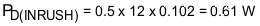Equation 29.Equation 30.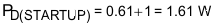From thermal shutdown limit graph at TA = 85°C, the shutdown time for 1.61 W power dissipation is ~1000 ms, which increases the margins further for shutdown time and ensures successful operation during start up and steady state conditions.

The spreadsheet tool available on the web can be used for iterative calculations.

#### 10.2.1.2.5 Support Component Selections - R4 and CIN

Reference to application schematics, R4 is required only if FLT is used; The resistor serves as pull-up for the open-drain output driver. The current sunk by this pin should not exceed 100 mA (refer to the Absolute Maximum Ratings table). CIN is a bypass capacitor to help control transient voltages, unit emissions, and local supply noise. Where acceptable, a value in the range of 0.001 μF to 0.1 μF is recommended for C(IN).

#### 10.2.1.3 Application Curves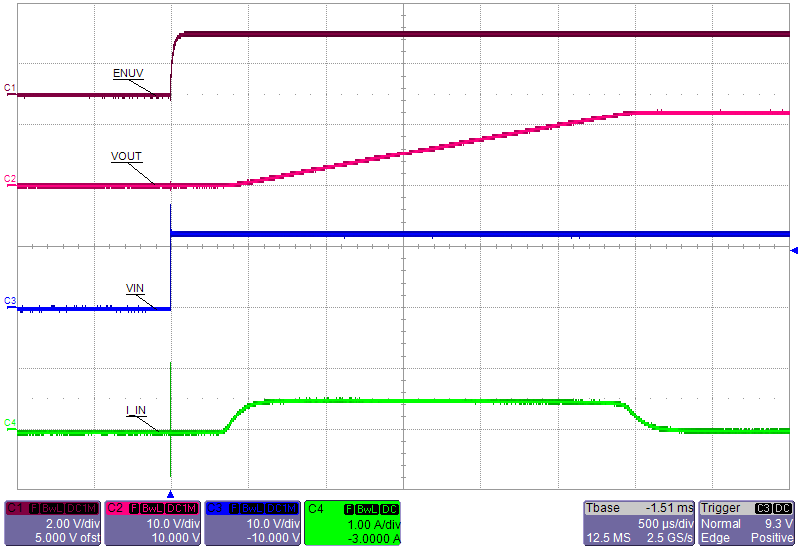Figure 36. Hot-Plug Start-Up: Output Ramp Without Load on Output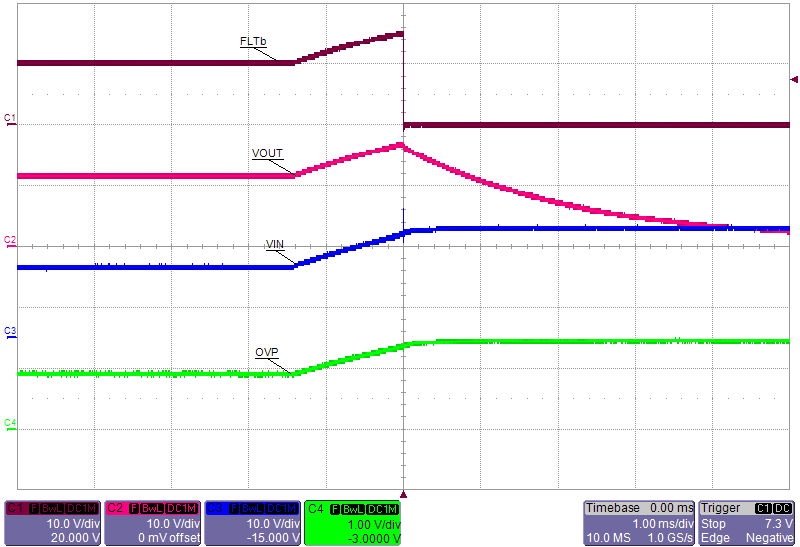Figure 38. Overvoltage Shutdown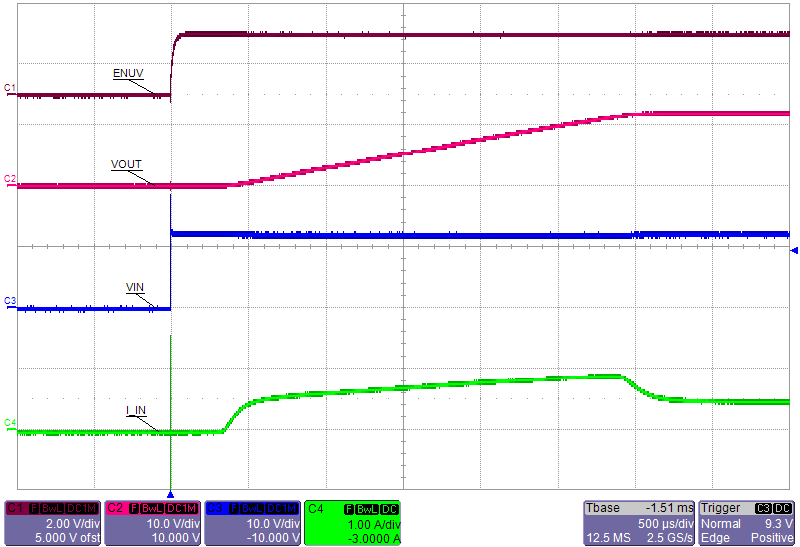Figure 37. Hot-Plug Start-Up: Output Ramp With 24 Ω Load at Start UpFigure 39. Overvoltage Recovery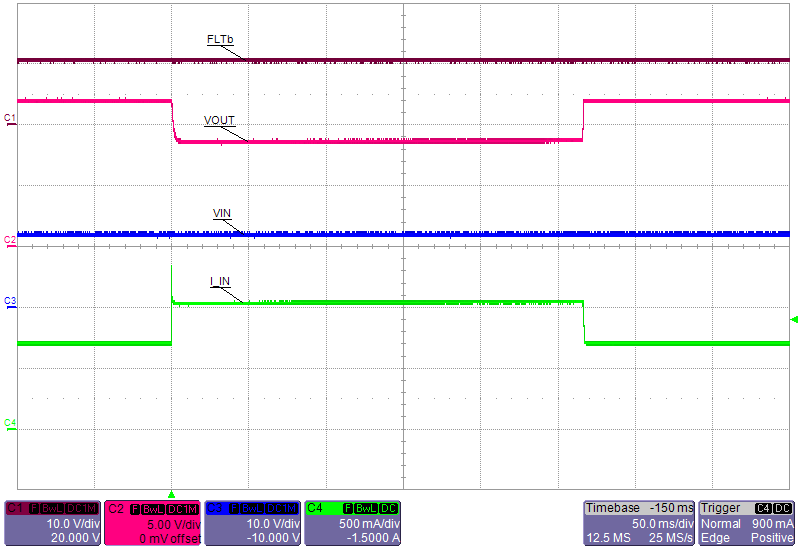Figure 40. Over Load: Step Change in Load from 19 Ω to
9 Ω Back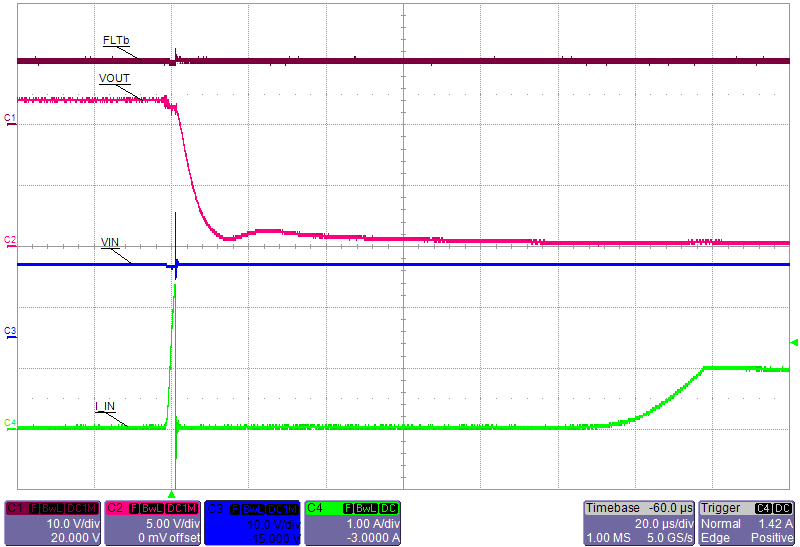Figure 42. Hot Short: Fast Trip and Current Regulation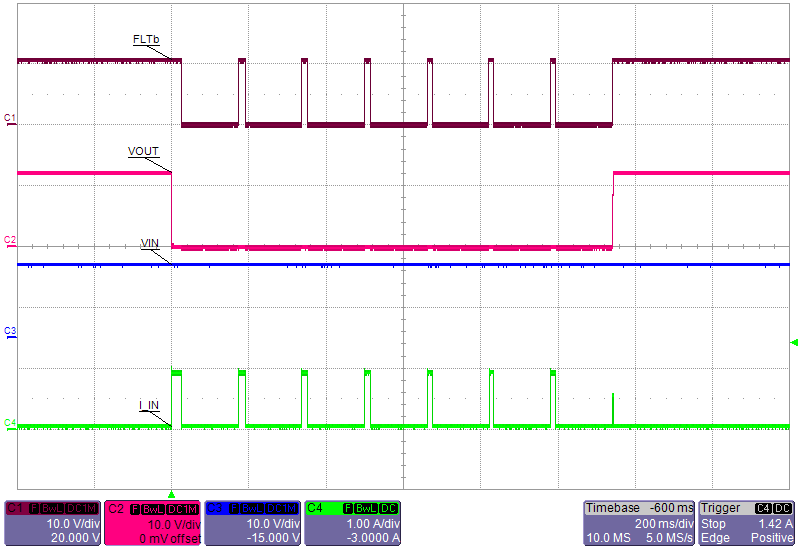Figure 44. Hot Short: Auto-Retry and Recovery from Short Circuit - TPS25921A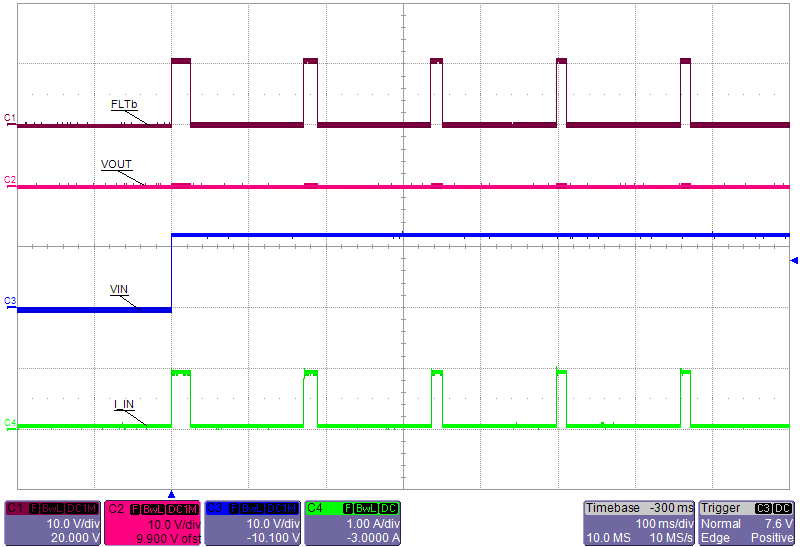Figure 46. Hot Plug-in with Short on Output: Auto-Retry - TPS25921A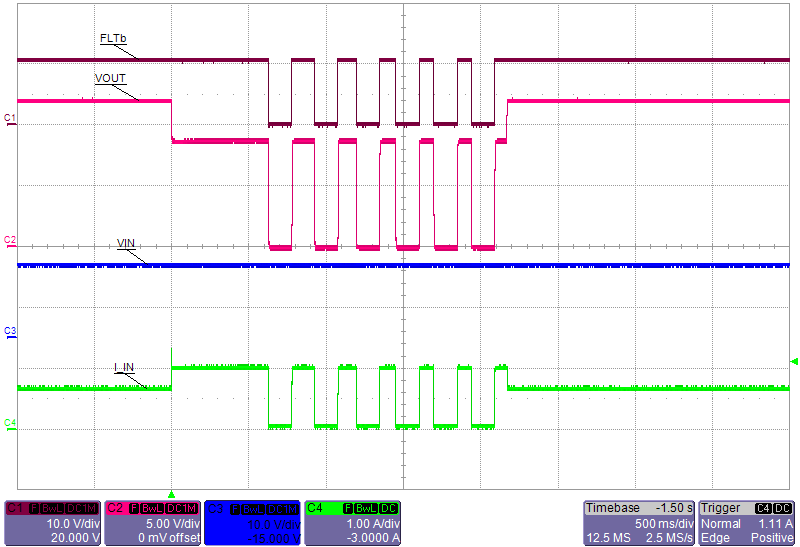Figure 41. Overload Condition: Auto Retry and Recovery - TPS25921A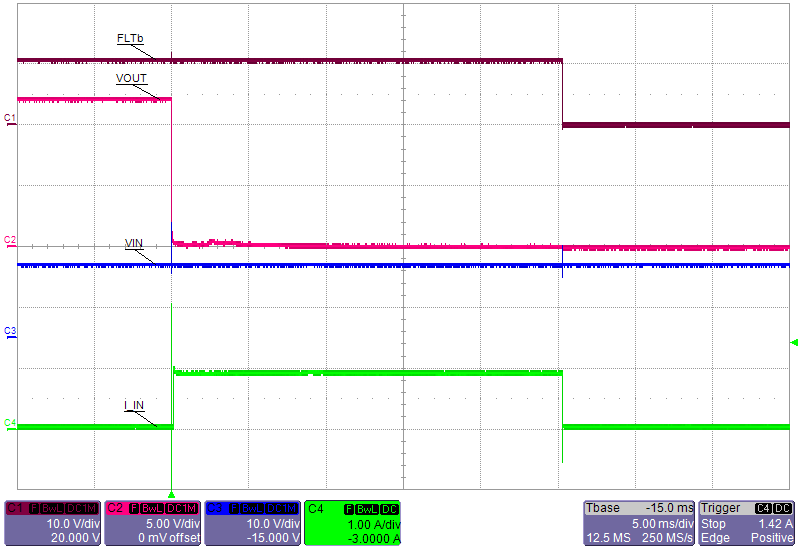Figure 43. Hot Short: Latched - TPS25921L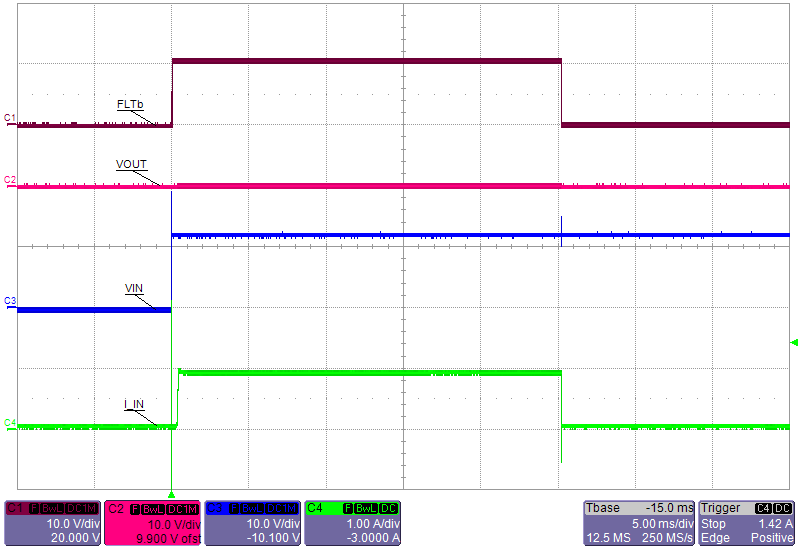Figure 45. Hot Plug-in with Short on Output: Latched - TPS25921L

### 10.3 System Examples

The TPS25921 provides a simple solution for current limiting, inrush current control and supervision of power rails for wide range of applications operating at 4.5 V to 18 V and delivering up to 1.5 A.

#### 10.3.1 Protection and Current Limiting for Primary-Side Regulated Power Supplies

Primary side regulated power supplies and adapters are dominant today in many of the applications such as Smart-phones, Portable hand-held devices, White Goods, Set-Top-Box and Gaming consoles. These supplies provide efficient, low cost and low component count solutions for power needs ranging from 5W to 30W. But, these come with drawbacks of

• No secondary side protection for immediate termination of critical faults such as short circuit and over voltage
• Do not provide precise current limiting for overload transients
• Have poor output voltage regulation for sudden change in AC input voltages - triggering output overvoltage condition

Many of the above applications require precise output current limiting and secondary side protection, driving the need for current sensing in the secondary side. This needs additional circuit implementation using precision operational amplifiers. This increases the complexity of the solution and also results in sensing losses The TPS25921 with its integrated low-ohmic N-channel FET provides a simple and efficient solution. Figure 47 shows the typical implementation using TPS25921.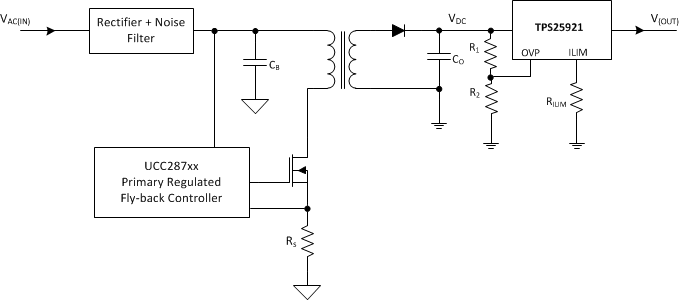Figure 47. Current Limiting and Protection for AC-DC Power Supplies

During short circuit conditions, the internal fast comparator of TPS25921 turns OFF the internal FET in less than 3 µs (typical) as soon as current exceeds I(FASTRIP), set by the current limit R(ILIM) resistor. The OVP comparator with 3% precision helps in quick isolation of the load from the input when inputs exceeds the set V(OVPR)

Figure 42 and Figure 38 shows short circuit and overvoltage response waveforms of implementation using TPS25921. In addition to above, the TPS25921 provides inrush current limit when output is hot-plugged into any of the system loads.

#### 10.3.2 Precision Current Limiting in Intrinsic Safety Applications

Intrinsic safety (IS) is becoming prominent need for safe operation of electrical and electronic equipment in hazardous areas. Intrinsic safety requires that equipment is designed such that the total amount of energy available in the apparatus is simply not enough to ignite an explosive atmosphere. The energy can be electrical, in the form of a spark, or thermal, in the form of a hot surface.

This calls for precise current limiting and precision shutdown of the circuit for over voltage conditions ensuring that set voltage and current limits are not exceeded for wide operating temperature range and variable environmental conditions. Applications such as Gas Analyzers, Medical equipment (such as electrocardiographs), Portal Industrial Equipment, Cabled Power distribution systems and hand-held motor operated tools need to meet these critical safety standards.

The TPS25921 device can be used as simple protection solution for each of the internal rails. Figure 48 shows the typical implementation using TPS25921.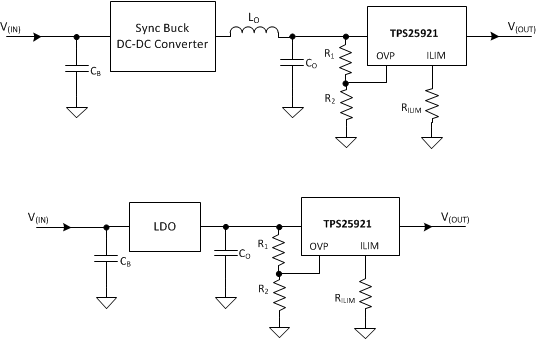Figure 48. Precision current Limit and Protection of Internal Rails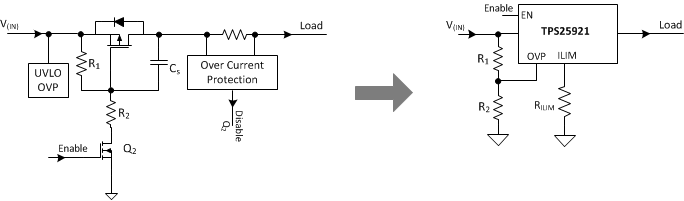Figure 49. Smart Load Switch Implementation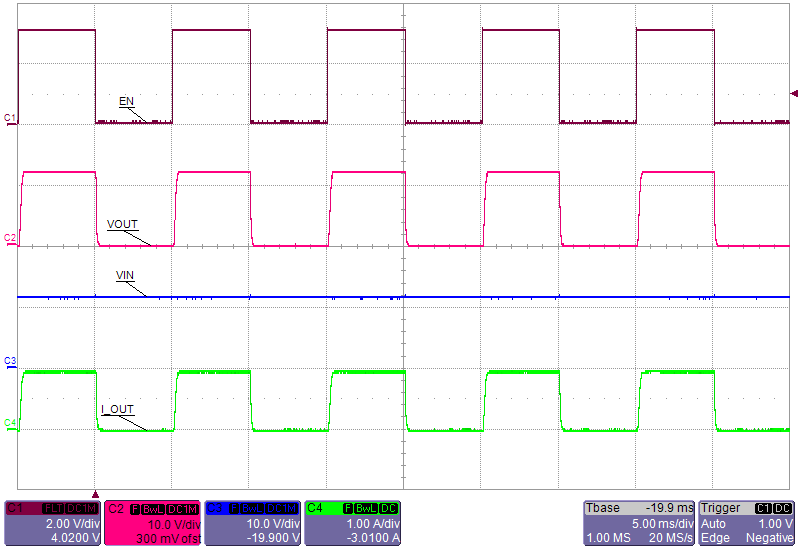Figure 50. Smart Load Switch (100 Hz Operation)